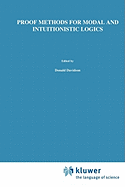Everywhere.

20% off your online order through 7/26! Use code: GETINK SEE DETAILS## Proof Methods For Modal And Intuitionistic Logics

&quot;Necessity is the mother of invention. &quot; Part I: What is in this book - details. There are several different types of formal proof procedures that logicians have invented. The ones we consider are: 1) tableau systems, 2) Gentzen sequent calculi, 3) natural deduction systems, and 4) axiom systems. We present proof procedures of each of these types for the most common normal modal logics: S5, S4, B, T, D, K, K4, D4, KB, DB, and also G, the logic that has become important in applications of modal logic to the proof theory of Peano arithmetic. Further, we present a similar variety of proof procedures for an even larger number of regular, non-normal modal logics (many introduced by Lemmon). We also consider some quasi-regular logics, including S2 and S3. Virtually all of these proof procedures are studied in both propositional and first-order versions (generally with and without the Barcan formula). Finally, we present the full variety of proof methods for Intuitionistic logic (and of course Classical logic too). We actually give two quite different kinds of tableau systems for the logics we consider, two kinds of Gentzen sequent calculi, and two kinds of natural deduction systems. Each of the two tableau systems has its own uses; each provides us with different information about the logics involved. They complement each other more than they overlap. Of the two Gentzen systems, one is of the conventional sort, common in the literature.

• Condition: --
• Format: Paperback
• Sold by: --
• Language: English
• Publisher: Springer Nature
• ISBN-13: 9789048183814
• ISBN: 9048183812
• Publication Year: 2010
• Edition: Reprint